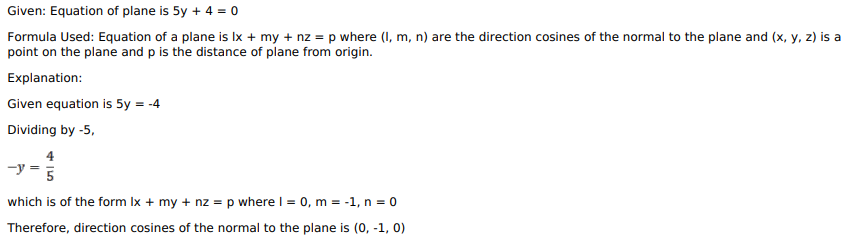# Mark against the correct answer in each of the following:Question:

Mark against the correct answer in each of the following:

The direction cosines of the normal to the plane $5 y+4=0$ are

A. $0, \frac{-4}{5}, 0$

B. $0,1,0$

C. $0,-1,0$

D. None of these

Solution: